# Lines and Angles

Go back to  'Geometry'

## Introduction to Angles

When two lines intersect at a point, the measure of the “opening” between these two lines is called an angle. Angles are usually measured in degrees.

Ever seen a game of football? Noticed where the players take the corner from? Well, the point at which the lines intersect is what forms an angle!## What is an angle?

Here is ray $$OB$$,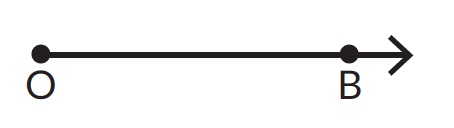There is another ray $$OA$$ that begins at $$O$$ and lies above $$OB$$. The "space" between $$OB$$ and $$OA$$ is called an angle. The angel's name is $$AOB$$, and is pronounced angle $$AOB$$, and written as \begin{align}\angle AOB \end{align}. It can also be called angle $$BOA$$—written out as \begin{align}\angle BOA \end{align}.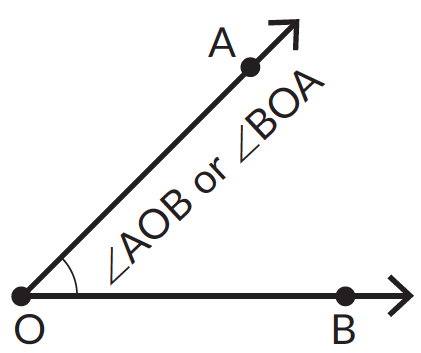There are two other ways of naming the angle that has been formed. One way is to simply use the name of the point where the angle is formed, which in this case is $$O.$$ This is written $$\angle O.$$ Another way is to use a number like 1 or 2 or 3 to name it. In mathematical shorthand, this is written $$\angle 1,\;\angle 2,\;\angle 3,\;$$ and so on. In this case, $$\angle AOB = \angle BOA = \angle O = \angle 1$$.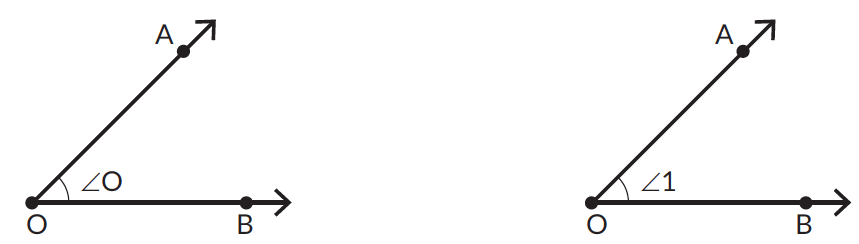## Types of Angles

### Right angle

An angle is measured in degrees. The symbol for degrees is ‘$$^\circ$$’. We defined an angle as the opening between two rays that begin from the same point. When there is no opening, that is, when the two rays lie on one another, the measure of the angle formed is zero degrees $$(0^\circ)$$. That is, \begin{align}\angle AOB = 0^\circ \end{align}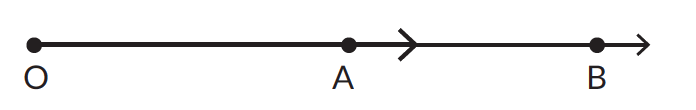The angle formed when a horizontal ray and a vertical ray meet is called a right angle. The measure of a right angle is  $$90^\circ$$. That is, \begin{align} \angle AOB =\,90^\circ\ \end{align}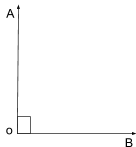Notice how the angle-bracket "ㄱ " that denotes a right angle is not curved like those used for other angles. This angle-bracket is used only to denote right angles. (However, it is not wrong to use the curved angle-bracket.)

### Straight angle

The angle formed by a straight line is called a straight angle. The measure of a straight angle is $$180^\circ$$. That is, \begin{align} \angle AOB =\,180^\circ\ \end{align}.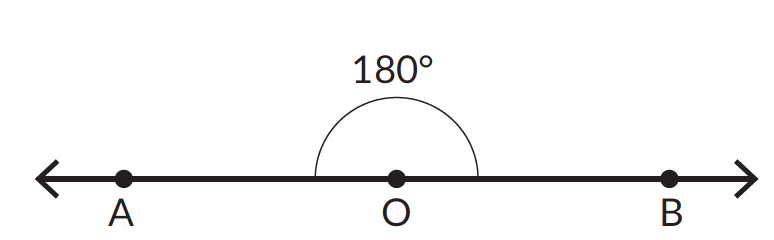### Acute angle

An acute angle is an angle whose measure is greater than $$0^\circ$$ and less than $$90^\circ$$, i.e., less than a right angle. For example, \begin{align} 0^\circ \lt \angle AOB \lt 90^\circ \end{align}.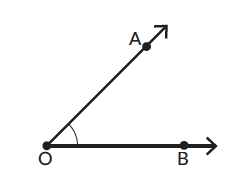### Obtuse angle

An obtuse angle is an angle whose measure is greater than $$90^\circ$$ and $$180^\circ$$, i.e., greater than a right angle and less than a straight angle. For example, \begin{align} 90^\circ \lt \angle PQR \lt 180^\circ \end{align}.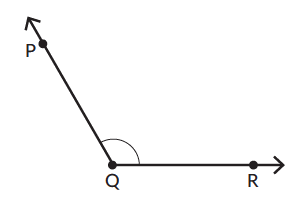### Reflex angle

A reflex angle is an angle whose measure is greater than $$180^\circ$$, i.e., greater than a straight angle and less than $$360^\circ$$, i.e., a full angle. For example, \begin{align} 180^\circ \lt \angle 3 \lt 360^\circ \end{align}.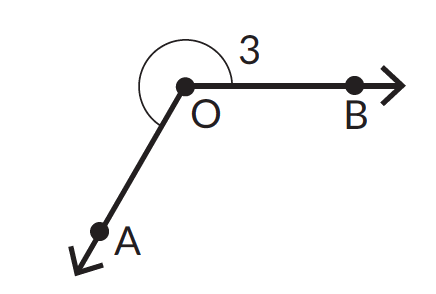### Complementary angles

When the sum of two angles is $$90^\circ$$, the angles are called complementary angles. Each angle is called the complement of the other angle.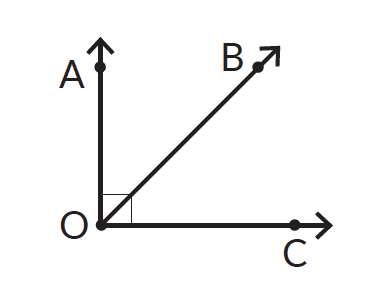\begin{align} \angle AOB + \angle BOC = \,90^\circ \end{align}. $$\angle AOB$$ and $$\angle BOC$$ are complementary angles.

### Supplementary angles

When the sum of two angles is $$180^\circ$$, the angles are called supplementary angles. Each angle is called the supplement of the other angle.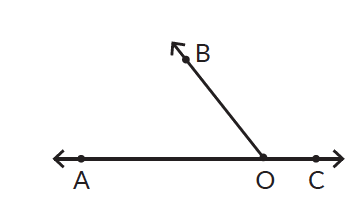\begin{align} \angle AOB + \angle BOC = \,180^\circ \end{align}. $$\angle AOB$$ and $$\angle BOC$$ are supplementary angles.

Any two angles that share a common ray, a common vertex, and whose interiors do not overlap are called adjacent angles.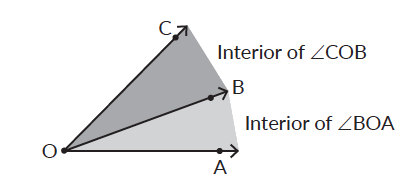$$\angle COB$$ and $$\angle BOA$$ have common vertex $$O$$. Their interiors don’t overlap and hence they are adjacent angles.

### Vertically opposite angles

The opposite angles formed by two intersecting lines are always equal. These angles are called vertically opposite angles. For e.g., in (b) $$\angle PTS$$ and $$\angle RTQ$$ are vertically opposite angles.## How to measure an angle?

Here is $$\angle AOB$$. We can tell by looking at it that it is acute, which means that its measure is greater than $$0^\circ$$ and less than $$90^\circ$$. What is its exact measure?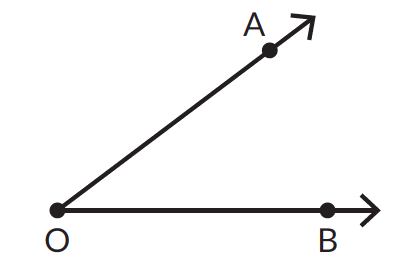We use the protractor to find out. We align the protractor with the ray $$OB$$ as shown. (Fig.1)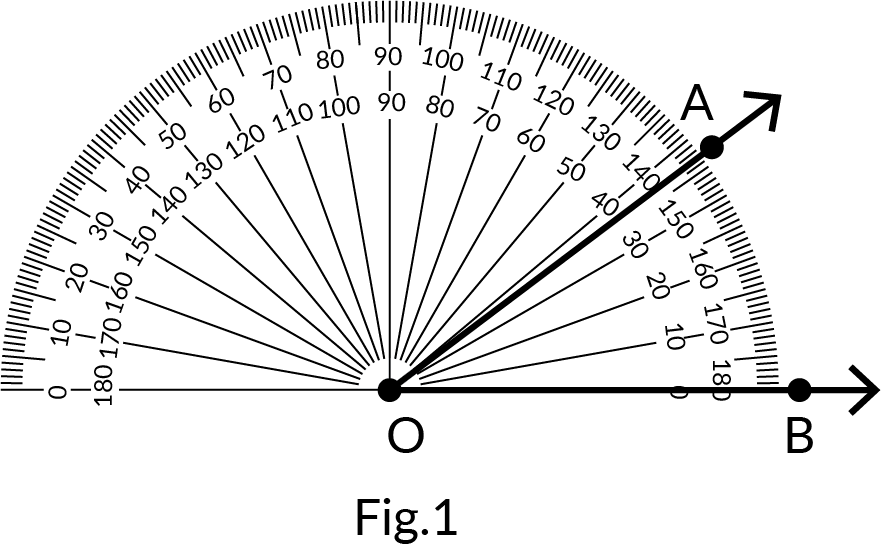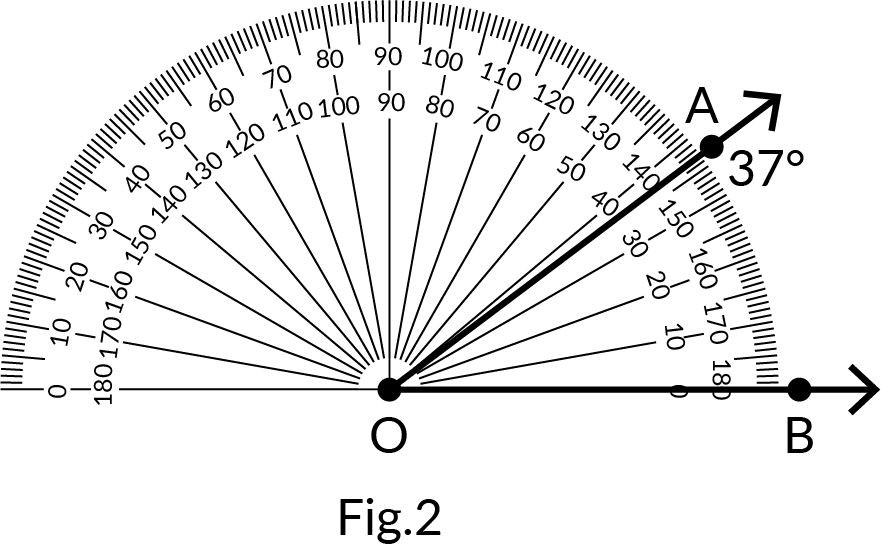Reading from the $$0^\circ$$ mark on the bottom-right of the protractor, the number on the protractor that coincides with the second ray is the measure of the angle. (Fig.2) Note that we measure the angle using the number on the "lower arc" of the protractor.

If the ray extends in the other direction (Fig.3) we measure the angle from the $$0^\circ$$ mark on the bottom-left. The number on the "top arc" of the protractor that coincides with $$OA$$ is the measure of $$\angle AOC$$. (Fig.4).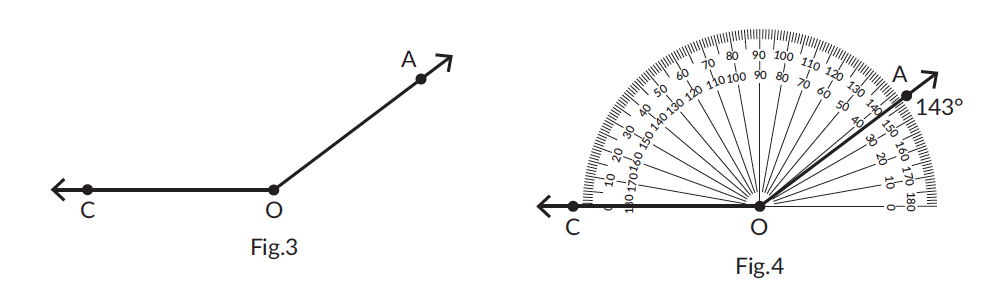(Note: If the ray, as drawn, is not long enough, extend it until it is possible to measure it with the protractor.)

## An angle and its reflex angle?

An angle is a "space" between two rays meeting at a common endpoint. It can be named using three letters.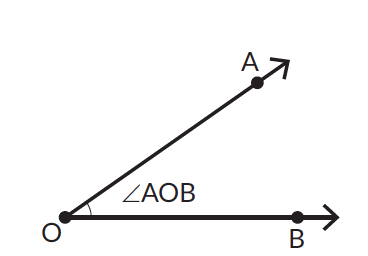But notice that writing $$\angle AOB$$ is not precise — while it usually refers to the non-reflex acute $$\angle AOB$$, it is possible for $$\angle AOB$$ to refer to reflex $$\angle AOB$$.It is to avoid this particular ambiguity that we refer to the angle marked above (with the dotted line) as reflex $$\angle AOB$$. Note how any $$\angle AOB$$ and its reflex $$\angle AOB$$ sum to $$360^\circ$$. This "property" can be used to measure (and construct) angles that measure more than $$180^\circ$$ and less than $$360^\circ$$.

## Procedure - How to construct angles?

We have used a protractor to measure angles. We will now use it to construct angles. Let’s draw a $$50^\circ$$ angle. We first draw a ray $$OB$$ and align the protractor with $$OB$$ as shown.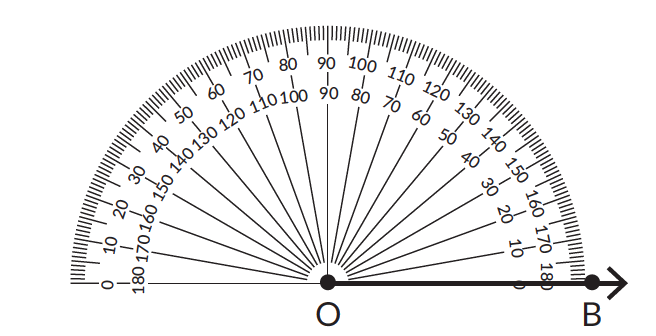We then place a point above the marking on the protractor that corresponds to $$50^\circ$$ (Fig.1). Then, we remove the protractor and draw a ray beginning at $$O$$ that passes through this point (Fig.2).

$$\angle AOB$$ is the required angle. That is \begin{align} \angle AOB = 50^\circ \end{align}.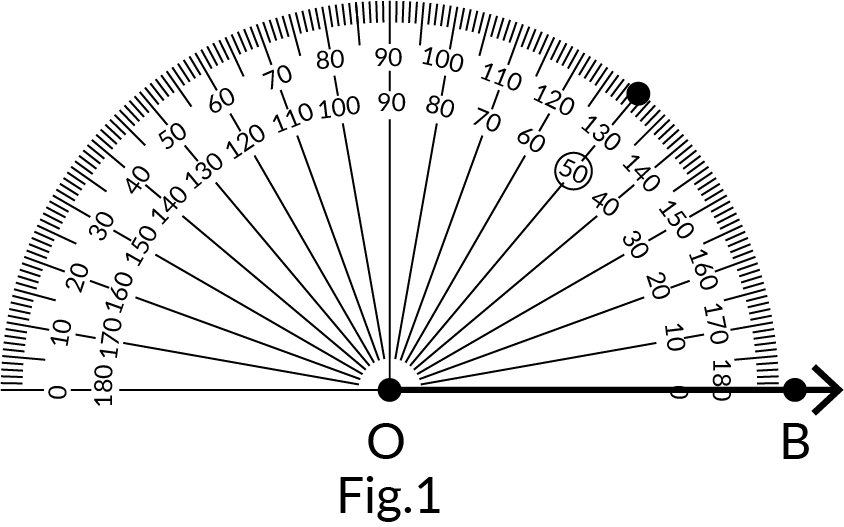Fig.3 and Fig.4 show how to draw a $$50^\circ$$ angle when the ray is pointing in the other direction.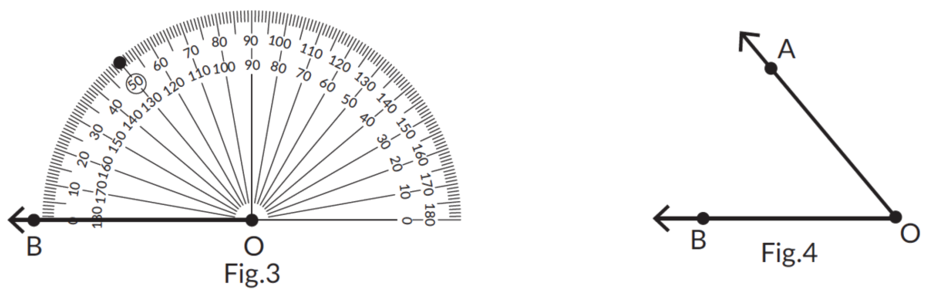## Types of angles (Grade 6)

### Transversal

Consider two lines, $$AB$$ and $$CD$$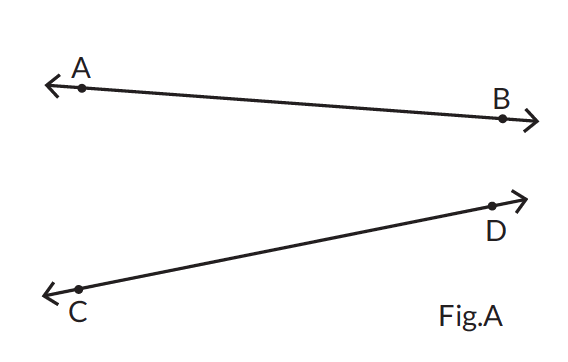Let line segment $$XY$$ be the line that intersects these two lines at two distinct points, $$P$$ and $$Q$$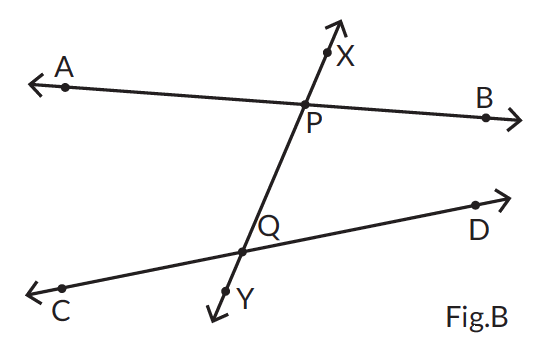A line that intersects two other lines at two distinct points is known as the transversal to the lines. In Fig.B, $$XY$$ is the transversal.

### Corresponding angles

Angles which have different vertices, lie on the same side of the transversal, and lie above (or below) the lines are called corresponding angles.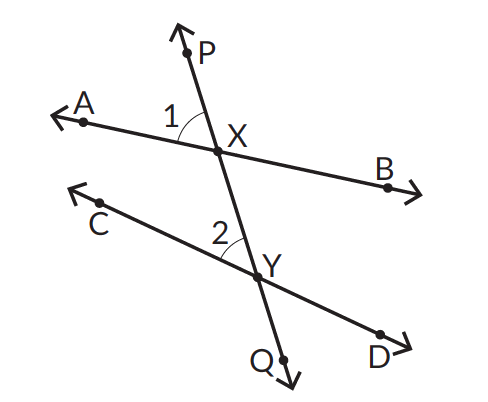In the given figure, line $$PQ$$ is the transversal. $$\angle 1$$ & $$\angle 2$$ have different vertices. They lie on the same side of the transversal. $$\angle 1$$ & $$\angle 2$$ both lie above the lines $$AB$$ and $$CD$$ respectively. Therefore $$\angle 1$$ & $$\angle 2$$ are corresponding angles.

When a transversal intersects two parallel lines, the corresponding angles formed are always equal.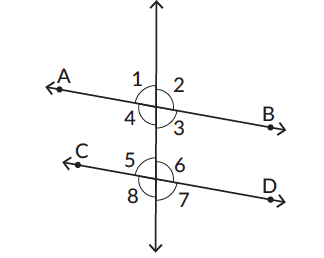$$\angle 1$$ & $$\angle 5$$, $$\angle 2$$ & $$\angle 6$$, $$\angle 4$$ & $$\angle 8$$, $$\angle 3$$ & $$\angle 7$$ are all pairs of corresponding angles.

### Alternate interior angles

Angles with different vertices, lying on the alternate sides of the transversal, and interior to the lines are called alternate-interior angles.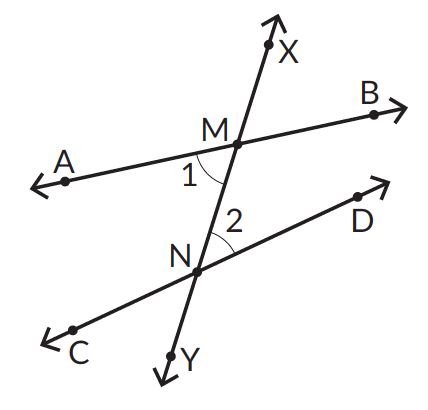In the given figure, line $$XY$$ is the transversal. $$\angle 1$$ & $$\angle 2$$ have different vertices. They lie on the opposite side of the transversal. $$\angle 1$$ & $$\angle 2$$ are angles interior to (in between) the lines $$AB$$ and $$CD$$ respectively. Therefore $$\angle 1$$ & $$\angle 2$$ are alternate-interior angles.

When a transversal intersects two parallel lines, the alternate interior angles formed are always equal.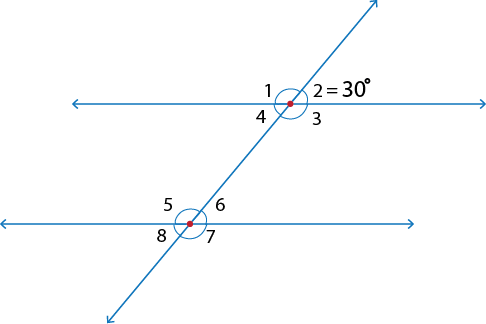\begin{align} \angle 4 = \, 30^\circ \end{align} (Hint: Vertically opposite angle.)
\begin{align} \angle 6 = \, 30^\circ \end{align} (Hint: Corresponding angle.)

The alternate interior angles $$\angle 4$$ & $$\angle 6$$ are equal.

### Alternate exterior angles

Angles with different vertices, lying on the alternate sides of the transversal, and exterior to the lines are called alternate-exterior angles.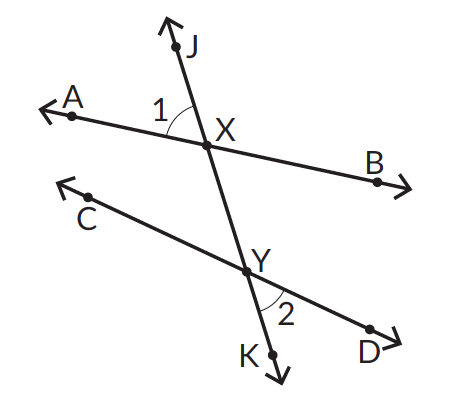In the given figure, line $$XY$$ is the transversal. $$\angle 1$$ & $$\angle 2$$ have different vertices. They lie on the opposite side of the transversal. $$\angle 1$$ & $$\angle 2$$ are angles exterior the lines $$AB$$ and $$CD$$ respectively. Therefore $$\angle 1$$ & $$\angle 2$$ are alternate-exterior angles.

When a transversal intersects two parallel lines, alternate exterior angles formed are always equal.\begin{align} \angle 4 = \, 30^\circ \end{align} (Hint: Vertically opposite angle.)
\begin{align} \angle 6 = \, 30^\circ \end{align} (Hint: Corresponding angle.)
\begin{align} \angle 8 = \, 30^\circ \end{align} (Hint: Vertically opposite angle.)

The alternate exterior angles $$\angle 2$$ & $$\angle 8$$ are equal.

### Co-interior angles

Angles with different vertices, lying on the same side of the transversal, and interior to the lines are called co-interior angles.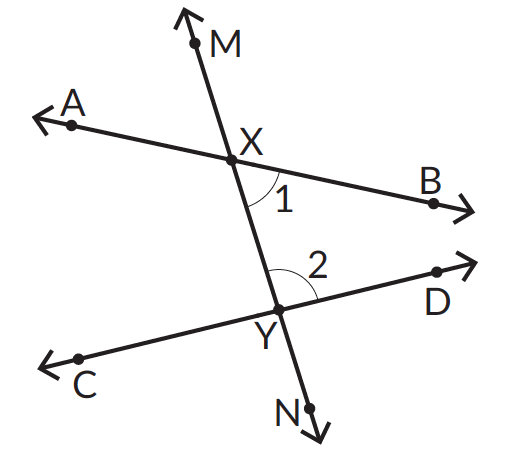In the given figure, line $$MN$$ is the transversal. $$\angle 1$$ & $$\angle 2$$ have different vertices. They lie on the same side of the transversal. $$\angle 1$$ & $$\angle 2$$ are angles interior to the lines $$AB$$ and $$CD$$ respectively. Therefore $$\angle 1$$ & $$\angle 2$$ are co-interior angles.

When a transversal intersects two parallel lines, the co-interior angles are always supplementary.\begin{align} \angle 4 = \, 30^\circ \end{align} (Hint: Vertically opposite angle)
\begin{align} \angle 3 = \, 150^\circ \end{align} (Hint: Supplementary angles)
\begin{align} \angle 6 = \, 30^\circ \end{align} (Hint: Corresponding angle)
\begin{align} \angle 5 = \, 150^\circ \end{align} (Hint: Supplementary angles)

\begin{align} \angle 4 + \angle 5 = \, 180^\circ \end{align}

### Exterior angle property of a triangle

Consider one of the exterior angles, \begin{align} \angle 4 \text { of } \triangle PQR \end{align}.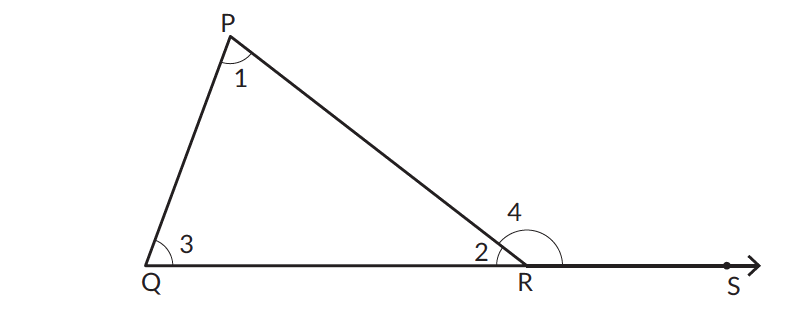In $$\triangle PQR$$, by the angle sum property,

\begin{align} & \angle 1 + \angle 2 + \angle 3 = \, 180^\circ \qquad \qquad \angle 3 + \angle 4 = \, 180^\circ \\ & \angle 1 + \angle 2 = \, 180^\circ - \angle 3 \qquad \qquad \angle 4 = \, 180^\circ - \angle 3 \end{align}

The sum obtained in both cases is equal,

\begin{align} & \angle 1 + \angle 2 = \, \angle 4 \end{align}

In any triangle, an exterior angle is always equal to the sum of its interior opposite angles. This is the exterior angle property of triangles.

## Tips and Tricks

• Tip - The easiest way to identify the angles is by looking at how wide or narrow is the ‘open space’. An acute angle has narrower space than a right angle. An obtuse angle has widest ‘open space’.
• Another easy way to identify the angles is by looking at the symbol given in the figure to show the angle type. For example, a right angle is always represented as shown.• In the picture given above $$\angle AOB$$ and $$\angle O$$ are the same. It is just different ways of denoting the same angle. Don’t get confused with it.
• While measuring the angles pay attention to the readings on the protractor. A protractor has 2 lines of markings this is so that you can measure the angle in any orientation. For an acute angle the reading is always less than $$90^\circ$$ and for an obtuse angle it is always more than $$90^\circ$$.

### Common mistakes or misconceptions

Misconception 1: Complementary angles have to be next to each other.

When the sum of two angles is $$90^\circ$$, the angles are called complementary angles. These angles need not be necessarily next to each other as long as their sum is $$90^\circ$$.In the image given above $$\angle ABC$$ and $$\angle PQR$$ are complementary angles. The sum of the two angles is $$90^\circ$$.

Misconception 2: $$\angle x$$ and reflex $$\angle x$$ are both one and the same.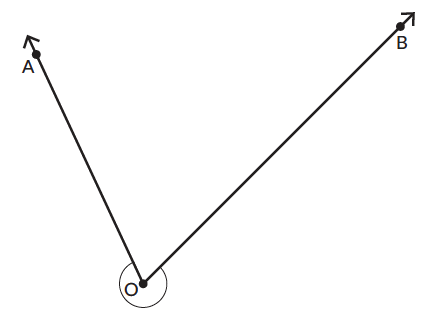In the image given above $$\angle PQR$$ is the non-reflex angle. Reflex $$\angle PQR$$ is the

Misconception 3: $$\angle x$$ and reflex $$\angle x$$ are both of the same degrees.

As the angles are different so is their measurement. The angles in all should sum up to $$360^\circ$$

Misconception 4: Any angles next to each other are adjacent angles.

Adjacent angles share a common side and vertex.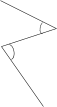The two angles don’t share a common side or a vertex and hence are not adjacent angles.

1. Name the angles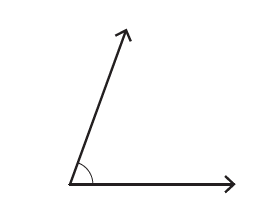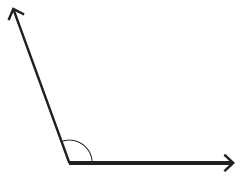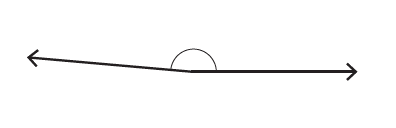Lines and Angles
Lines and Angles
Lines and Angles
Lines and Angles
Measuring and Constructing Angles
Measuring and Constructing Angles